Courses

# Position, Path length,Displacement and Speed and Velocity (Average and Instantaneous) Class 11 Notes | EduRev

## Class 11 : Position, Path length,Displacement and Speed and Velocity (Average and Instantaneous) Class 11 Notes | EduRev

The document Position, Path length,Displacement and Speed and Velocity (Average and Instantaneous) Class 11 Notes | EduRev is a part of the Class 11 Course Physics For JEE.
All you need of Class 11 at this link: Class 11

Position
Position can be understood as the location of an object with respect to a reference point. It is expressed as a position vector.
Shown below is the position of a cyclist with respect to a lamp post. Cyclist starts at the lamp post and goes to Position 1, 2, 3 and 4 respectively.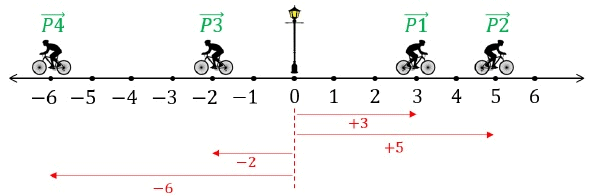Position 1, P1→ = +3 units
Position 2, P2→ = +5 units
Position 3, P3→ = -2units
Position 4, P4→ = -6units
Path Length/Distance

• The total length of the path covered by the moving object.
• It is dependent on the path chosen, thus for motion between two fixed points A and B we can have many different values of the distance traversed.
• It is a scalar quantity, as the length of the path has no indication of the direction in it.
• Its SI unit is meter (m) and its dimension is (L).
• The following image shows the distance covered by the same cyclist.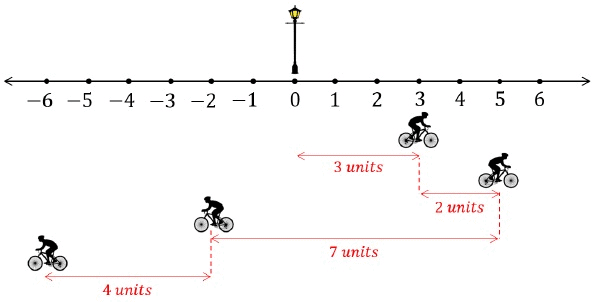Path Length = 16 units = Distance

Displacement

• Shortest distance between the initial and final positions. It is a vector.
• Its magnitude is the minimum distance between the final and initial point and is directed from the initial position to the final position.
• For a particle moving along the x-axis, motion from one position x1 to another position x2, is displacement, Δx where,
Δx = x- x1
• If the particle moves from x1= 4m to x= 12 m, then Δx = (12m)-(4m) = +8m. The positive result indicates that the motion is in the positive direction. If the particle then returns to x = 4m, the displacement for the full trip is zero. The actual number of meters covered for the full trip is irrelevant, displacement involves only the original and final position.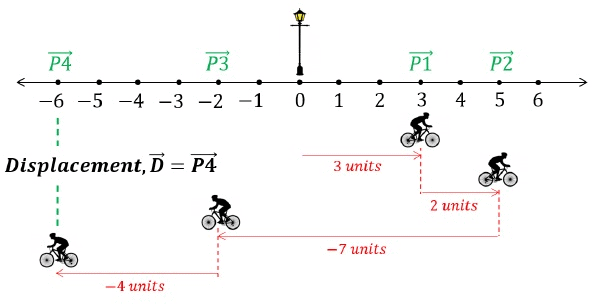Final Displacement, D→ = -6 units

It is interesting to observe that the final displacement vector is the same as the final position vector. This is because,

P1→ + P2→ + P3→ + P4→
D→ = 3 + 2 - 7 - 4 = -6 units

General Formula of Displacement

Displacement between two positions starting at PA→ and ending at PB→ is defined as −

Displacement, D→ = PB→ - PA→

For Example, in the figure below,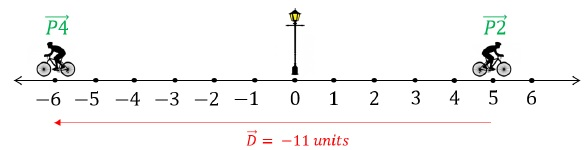Displacement between position 2 and position 4,

D→ = P4→ - P2→ = -6 - (+5) = -11 units

Distance and Displacement Relationship

The magnitude of Displacement may or may not be equal to Distance.

For example, in the figure below,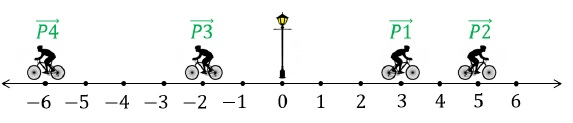Between P1→ and P2→ −

Distance = 2 units

Magnitude of displacement = |+5 - (+3)| = 2 units

Between P1→ and P3→ −

Distance = 9 units

Magnitude of displacement = |-2 - (+3)| = 5 units

Important Note:

• If the object doesn’t change direction during motion, distance is equal to magnitude of displacement. (as evident between P1→ and P2→)
• If the object changes direction during motion, distance is always greater than displacement. (as evident between P1→ and P3→)

Offer running on EduRev: Apply code STAYHOME200 to get INR 200 off on our premium plan EduRev Infinity!

## Physics For JEE

187 videos|516 docs|263 tests

,

,

,

,

,

,

,

,

,

,

,

,

,

,

,

,

,

,

,

,

,

,

,

,

,

,

,

;